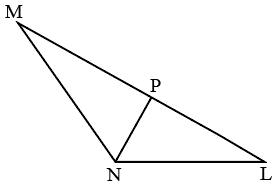Question 5

# In $$\triangle MNL$$,line NP bisects the angle MNL. If NP : NL=2: 3 and angle MNL=$$120^\circ$$. Then NP: NL: MN is:Solution

Let the value of MN be p

Since NP is the angular bisector

NP= $$\ \frac{\ 2\times\ MN\times\ NL}{MN+NL}\cos\theta\ \$$

2x=$$\ \frac{\ 2\times\ p\times\ 3x}{p+3x}\cos60$$

p=6x

NP: NL: MN = 2:3:6

Derivation for the length of angular bisector:

Let CP be the angular bisector of $$\angle\ ACB$$. And lengths of AB,BC,CA, CP,AP,PB be c,a,b,x,m,n respectively.

From triangles CPB and CPA using cosine rule we get:

$$n^2=a^2+x^2-2axCos\left(C\right)\ and\ m^2=b^2+x^2-2bxCos\left(C\right)$$

According to angle biscetor rule: $$\ \frac{b}{m}=\frac{a}{n}$$

.'.$$\frac{a^2}{b^2}=\frac{n^2}{m^2}$$= $$\frac{a^2+x^2−2axCos(C)}{\left(b^2+x^2−2bxCos(C\right)}$$.

$$\frac{a^2}{b^2}=\frac{a^2+x^2−2axCos(C)​}{b^2+x^2-2bxCos\left(C\right)}$$

Upon equating we get, $$(a^2−b^2)x−2ab(a−b)Cos(C)=0$$

since x$$\ne\ 0$$,

x=$$\frac{2ab(a−b)Cos(C)}{a^2−b^2}$$

.'.x=$$\frac{2abCos(C)}{a+b}$$.

• All Quant Formulas and shortcuts PDF
• 40+ previous papers with solutions PDF

##### urooj fatima

2 years, 6 months ago

there is a better way. please upload another way. this is formula based. and that too a formula rarely known to aspirants. Plz cracku dont make fun of us.

##### suresh chand

1 year, 5 months ago

Yes

OR